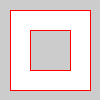# begin_contour()#

Use the begin_contour() and end_contour() methods to create negative shapes within shapes such as the center of the letter ‘O’.

## Examples#def setup():
py5.translate(50, 50)
py5.stroke(255, 0, 0)
py5.begin_shape()
# exterior part of shape, clockwise winding
py5.vertex(-40, -40)
py5.vertex(40, -40)
py5.vertex(40, 40)
py5.vertex(-40, 40)
py5.begin_contour()
# interior part of shape, counter-clockwise winding
py5.vertex(-20, -20)
py5.vertex(-20, 20)
py5.vertex(20, 20)
py5.vertex(20, -20)
py5.end_contour()
py5.end_shape(py5.CLOSE)def setup():
py5.translate(50, 50)
py5.stroke(255, 0, 0)
with py5.begin_closed_shape():
# exterior part of shape, clockwise winding
py5.vertex(-40, -40)
py5.vertex(40, -40)
py5.vertex(40, 40)
py5.vertex(-40, 40)
with py5.begin_contour():
# interior part of shape, counter-clockwise winding
py5.vertex(-20, -20)
py5.vertex(-20, 20)
py5.vertex(20, 20)
py5.vertex(20, -20)


## Description#

Use the begin_contour() and end_contour() methods to create negative shapes within shapes such as the center of the letter ‘O’. The begin_contour() method begins recording vertices for the shape and end_contour() stops recording. The vertices that define a negative shape must “wind” in the opposite direction from the exterior shape. First draw vertices for the exterior shape in clockwise order, then for internal shapes, draw vertices counterclockwise.

These methods can only be used within a begin_shape() & end_shape() pair and transformations such as translate(), rotate(), and scale() do not work within a begin_contour() & end_contour() pair. It is also not possible to use other shapes, such as ellipse() or rect() within.

This method can be used as a context manager to ensure that end_contour() always gets called, as shown in the second example.

Underlying Processing method: beginContour

## Signatures#

begin_contour() -> None


Updated on March 06, 2023 02:49:26am UTC